# Combinatorial number + sets - math problems

#### Number of problems found: 8

• Subsets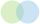How many 19 element's subsets can be made from the 26 element set?
• Raffle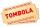There are 200 draws in the raffle, but only 20 of them win. What is the probability of at least 4 winnings for a group of people who have bought 5 tickets together?
• Pairs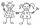From the five girls and four boys teachers have to choose one pair of boy and girl. A) How many such pairs of (M + F)? B) How many pairs where only boys (M + M)? C) How many are all possible pairs?
• Combinations 66 purses 9 flaps 12 straps Every combination must include 1 purse, 1 flap, and 1 strap. How many are possible combinations?
• DeficienciesDuring the hygienic inspection in 2000 mass caterers, deficiencies were found in 300 establishments. What is the probability that deficiencies in a maximum of 3 devices will be found during the inspection of 10 devices?
• Math logicThere are 20 children in the group, each two children have a different name. Alena and John are among them. How many ways can we choose 8 children to be among the selected A) was John B) was John and Alena C) at least one was Alena, John D) maximum one wa
• Hazard game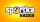In the Sportka hazard game, 6 numbers out of 49 are drawn. What is the probability that we will win: a) second prize (we guess 5 numbers correctly) b) the third prize (we guess 4 numbers correctly)?
• Three students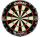Three students independently try to solve the problem. The first student will solve a similar problem with a probability of 0.6, the second student will solve at a probability of 0.55, and the third will solve at a probability 0.04. The problem is resolve

We apologize, but in this category are not a lot of examples.
Do you have an interesting mathematical word problem that you can't solve it? Submit a math problem, and we can try to solve it.

We will send a solution to your e-mail address. Solved examples are also published here. Please enter the e-mail correctly and check whether you don't have a full mailbox.

Please do not submit problems from current active competitions such as Mathematical Olympiad, correspondence seminars etc...

See also our combinations calculator. Combinatorial number - math word problems. Sets - math word problems.